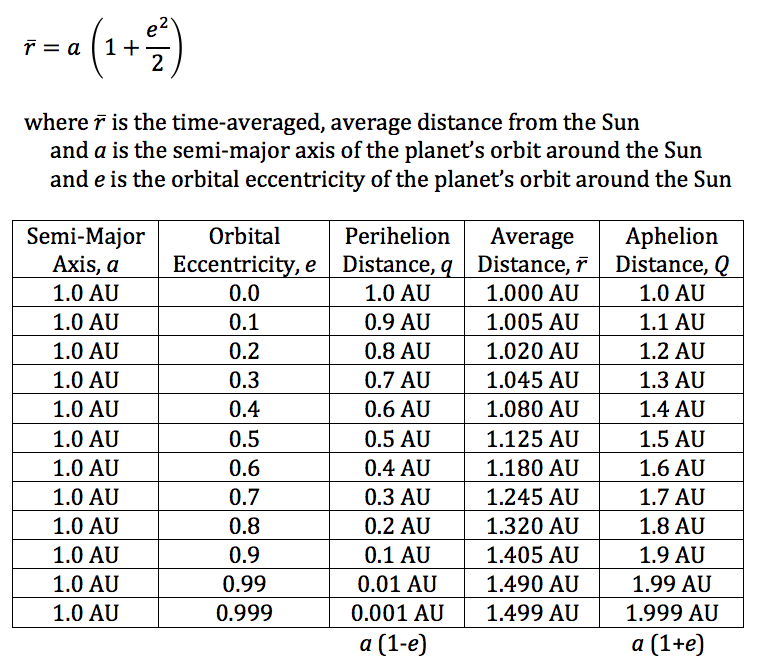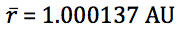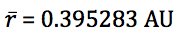# Average Orbital Distance

If a planet is orbiting the Sun with a semi-major axis, a, and orbital eccentricity, e, it is often stated that the average distance of the planet from the Sun is simply a.  This is only true for circular orbits (e = 0) where the planet maintains a constant distance from the Sun, and that distance is a.

Let’s imagine a hypothetical planet much like the Earth that has a perfectly circular orbit around the Sun with a = 1.0 AU and e = 0.  It is easy to see in this case that at all times, the planet will be exactly 1.0 AU from the Sun.

If, however, the planet orbits the Sun in an elliptical orbit at a = 1 AU and e > 0, we find that the planet orbits more slowly when it is farther from Sun than when it is nearer the Sun.  So, you’d expect to see the time-averaged average distance to be greater than 1.0 AU.  This is indeed the case.The Earth’s current osculating orbital elements give us:

a = 0.999998 and e = 0.016694

Earth’s average distance from the Sun is thus:Mercury, the innermost planet, has the most eccentric orbit of all the major planets:

a = 0.387098 and e = 0.205638

Mercury’s average distance from the Sun is thus: